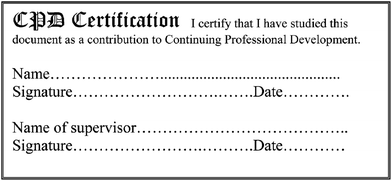# Using the Grubbs and Cochran tests to identify outliers

Analytical Methods Committee, AMCTB No. 69

First published on 30th July 2015

In a previous Technical Brief (TB No. 39) three approaches for tackling suspect results were summarised. Median-based and robust methods respectively ignore and down-weight measurements at the extremes of a data set, while significance tests can be used to decide if suspect measurements can be rejected as outliers. This last approach is perhaps still the most popular one, and is used in several standards, despite possible drawbacks. Here significance testing for identifying outliers is considered in more detail with the aid of some typical examples.

Significance tests can be used with care and caution to help decide whether suspect results can be rejected as genuine outliers (assuming that there is no obvious explanation for them such as equipment or data recording errors), or must retained in the data set and included in its later applications. Such tests for outliers are used in the conventional way, by establishing a null hypothesis, H0, that the suspect results are not significantly different from the other members of the data set, and then rejecting it if the probability of obtaining the experimental results turns out to be very low (e.g. p < 0.05). If H0 can be rejected the suspect result[s] can also be rejected as outliers. If H0 is retained the suspect results must be retained in the data set. These situations are often distinguished by converting the experimental data into a test statistic and comparing the latter with critical values from statistical tables. If the two are very similar, i.e. the suspect result is close to the boundary of rejection or acceptance, the test outcome must be treated with great circumspection.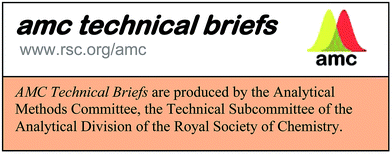## Suspect results in replicate measurements

Fig. 1 shows as a dot plot the results obtained when the cholesterol level in a single blood serum sample was measured seven times. The individual measurements are 4.9, 5.1, 5.6, 5.0, 4.8, 4.8 and 4.6 mM. The dot plot suggests that the result 5.6 mM is noticeably higher than the others: can it be rejected as an outlier? This decision might have a significant effect on the clinical interpretation of the data. Several tests are available in this situation. For years the most popular was the Dixon or Q-test, introduced in 1951. It has the advantage that (as in this example) the test statistic can often be calculated mentally. It has been superseded as the recommended method (ISO 17025) by the Grubbs test (1950), which compares the difference between the suspect result and the mean of all the data with the sample standard deviation. The test statistic, G, in this simplest case is thus given by:
 G = |suspect value −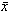|/s (1)
where the sample mean and standard deviation,and s, are calculated with the suspect value included. In our exampleand s are 4.97 and 0.32 respectively, so G = 0.63/0.32 = 1.97, which is less than the two-tailed critical value (p = 0.05) of 2.02. We conclude that the suspect value, 5.6 mM, is not an outlier, and must be included in any subsequent application of the data (the Dixon test leads to the same conclusion). One important lesson of this example is that in a small data sample a single suspect measurement must be very different from the rest before it qualifies for rejection as outlier. In this instance the Grubbs and Dixon tests agree that only if the suspect measurement is as high as 5.8 mM can it be (just) rejected (Fig. 1). Both these tests suffer from drawbacks. The more important one is that they assume that the data sample is drawn from a normally distributed population. This is a reasonable assumption in this example and other simple situations, but in other cases it might be a risky supposition. A measurement that seems to be an outlier assuming a normal population distribution might well not be an outlier if the distribution is, for example, log-normal. Serum cholesterol levels across the population are not normally distributed, so if the values above came from different individuals it would be quite inappropriate to test the value 5.6 mM as a possible outlier.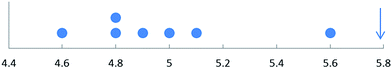Fig. 1 Dot-plot for serum cholesterol data (mM). The arrow marks the value that the highest measurement would have to take before it could just be regarded as an outlier.

A separate practical issue arises if the data set contains two or more suspect results: these may all be at the high end of the data range, all at the low end, or at both ends of the range. The Grubbs method can be adapted to these situations. If two suspect values, x1 and xn, occur at opposite ends of the data set, then G is simply given by:

 G = (xn − x1)/s (2)

In eqn (1) and (2) the value of G evidently increases as the suspect values become more extreme, so G values greater than the critical values allow the rejection of H0. When there are two suspect values at the same end of the data set two separate standard deviations must be calculated: s is the standard deviation of all the data, and s′ is the standard deviation of the data with the two suspect values excluded. G is then given by:

 G = (n − 3)s′2/(n − 1)s2 (3)

In this case as the pair of suspect values becomes more extreme s2, and hence also G, becomes smaller. So G values smaller than the critical ones allow the rejection of H0. Inevitably the critical values used in conjunction with eqn (1)–(3) are different. The Grubbs tests can be used sequentially: if a single outlier is not detected using eqn (1), then the other tests can be used to ensure that one outlier is not being masked by another.

Many widely available suites of statistical software provide facilities for implementing the Grubbs test, and spreadsheets can also be readily modified to give G values. Critical values for G are given in the reference below.

## Suspect results in analysis of variance

A separate test for outliers of a different kind is the Cochran test, introduced as long ago as 1941, which can be applied when Analysis of Variance (ANOVA) is used to study the results of collaborative trials (method performance studies). In such trials the overall variance observed, i.e. the reproducibility variance, is taken to be the sum of the repeatability variance, i.e. that due to measurement error, and the variance reflecting genuine between-laboratory differences. This relationship assumes that at a given analyte concentration the repeatability variance is the same in all laboratories. Though all such trials involve the use of the same analytical method in all the laboratories, this assumption is not necessarily valid, and in practice substantial differences in repeatability variance may be evident. Results from a laboratory with a suspect repeatability variance can then be excluded (ISO 5725-2) if it is shown by the Cochran test to be an outlier. The test statistic, C, is given by: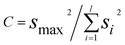(4)
where smax2 is the suspect repeatability variance and the si2 values are the variances from all the l participating laboratories. Some collaborative trials utilise only two measurements at each concentration of the analyte in each laboratory, and the equation then simplifies to: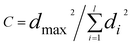(5)
where the di values are the differences between the pairs of results. A value of C that is greater than the critical value allows the result from the laboratory in question to be rejected. Critical values of C at a given probability level depend on the number of measurements made in each laboratory at a given concentration, and the number of participating laboratories. Sometimes the laboratories may make slightly different numbers of measurements on a given material, in which case the average number of measurements is used to provide the critical value of C. Of course the Cochran test can be used with any other applications of ANOVA where it is desirable to test the assumption of homogeneity of variance: it has been applied to unbalanced ANOVA designs, and adapted to allow it to be applied sequentially if more than one suspect variance occurs. The test has also been used in process control studies and in time series analysis. Critical values of C are given in the reference below.

## Conclusions

The Grubbs and Cochran tests are frequently used in tandem in evaluating the results of collaborative trials. The normal sequence is that the Cochran test is first applied to any suspect repeatability variances, with the Grubbs test next applied to single and then multiple suspect mean measurement values. (Note: procedures defined in ISO 5275 and IUPAC for carrying out this sequence differ somewhat.) In practice, however, it is likely that the data from such trials are likely to be normally distributed, but contaminated with a small number of erroneous or extreme measurements. These are the ideal conditions for the application of robust statistical methods, and information and software for several of these, including robust ANOVA calculations, are available from the Royal Society of Chemistry's website.

James N. Miller.

This Technical Brief was prepared by the Statistical Subcommittee, and approved by the Analytical Methods Committee on 15/06/15.